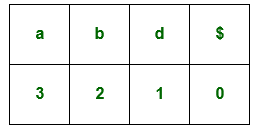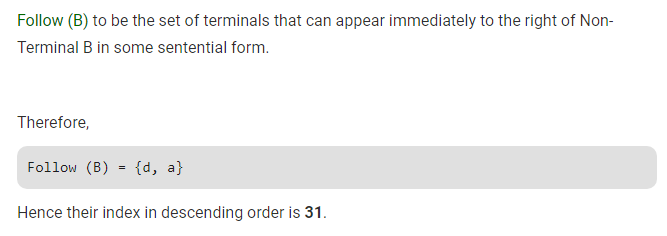# Gate CS-2019 Question Paper With Solutions

Q. 28 Consider the following given grammar:

```S → Aa
A → BD
B → b|ε
D → d|ε```

Let a, b, d and \$ be indexed as follows:Compute the FOLLOW set of the non-terminal B and write the index values for the symbols in the FOLLOW set in the descending order. (For example, if the FOLLOW set is {a, b, d, \$}, then the answer should be 3210).

Note: This was Numerical Type question.

(A) 31

(B) 310

(C) 230

(D) 23